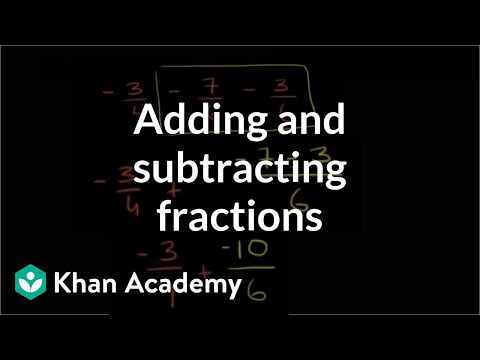Video

# Substitution with negative numbers (Full video)

Description: Practice substituting positive and negative values for variables. Let's give ourselves some practice substituting positive and negative values for variables. So we're told to evaluate X, we're told to evaluate X minus negative Y, where X is equal to negative two and Y is equal to five. So it's going to be negative two plus five, which is equal to three.

### Other videos you might be interested in### Absolute value as distance between numbers (Full video)